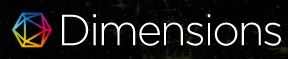### SOME PROPERTIES OF COPRIME GRAPH OF DIHEDRAL GROUP D_2n WHEN n IS A PRIME POWER

Abdul Gazir S, I Gede Adhitya Wisnu Wardhana, Ni Wayan Switrayni, Qurratul Aini

DOI: https://doi.org/10.14710/jfma.v3i1.7413

#### Abstract

The Study of algebraic structures, especially on graphs theory, leads to a
new topics of research in recent years. In this paper, the algebraic structures that will be represented by a coprime graph are the dihedral group and its subgroups. The coprime graph of a group G, denoted by \Gamma_D_2n is a graph whose vertices are elements of G and two distinct vertices a and b are adjacent if only if (|a,|b|)=1. Some properties of the coprime graph of a dihedral group D_2n are obtained. One of the results is if n is prime then \Gamma_D_2n is a complete bipartite graph. Moreover, if n is the power of prime then \Gamma_D_2n is a multipartite graph.

PDF

#### References

X.L. Ma, H.Q. Wei, and L.Y. Yang, ”The coprime graph of a group,” International Journal of Group Theory, vol. 3, no. 3, pp. 13–23, 2014.

R. Munir, Matematika Diskrit. Bandung: Penerbit Informatika, 2010.

H.M. Dorbidi, ”A note on the coprime graph of a group,” International Journal of Group Theory, vol. 5, no. 4, pp. 17–22, 2016.

J. B. Fraleigh, A First Course in Abstract Algebra. Harlow: Pearson New International Edition, 2014.

A. Gazir S and I.G.A.W. Wardhana, ”Subgrup Non Trivial Dari Grup Dihedral,” Eigen Mathematics Journal, vol. 2, no. 2, pp. 73–76, 2019.

### Refbacks

• There are currently no refbacks.

### Publisher:

Department of Mathematics, Faculty of Science and Mathematics, Diponegoro University

Mailing address: Jl. Prof Soedarto, SH, Tembalang, Semarang, Indonesia 50275

Telp./Fax             : (+6224) 70789493 / (+62224) 76480922

Website              : www.math.fsm.undip.ac.id